Courses

# Test: Frequency Response

## 20 Questions MCQ Test Electronic Devices | Test: Frequency Response

Description
This mock test of Test: Frequency Response for Electrical Engineering (EE) helps you for every Electrical Engineering (EE) entrance exam. This contains 20 Multiple Choice Questions for Electrical Engineering (EE) Test: Frequency Response (mcq) to study with solutions a complete question bank. The solved questions answers in this Test: Frequency Response quiz give you a good mix of easy questions and tough questions. Electrical Engineering (EE) students definitely take this Test: Frequency Response exercise for a better result in the exam. You can find other Test: Frequency Response extra questions, long questions & short questions for Electrical Engineering (EE) on EduRev as well by searching above.
QUESTION: 1

### (Q.1-Q.3) A parallel resonant circuit has a resistance of 2k ohm and half power frequencies of 86 kHz and 90 kHz. Q. The value of capacitor is

Solution:

BW = 2p(90-86)k = 1/RC or C = 19.89 nF.

QUESTION: 2

### The value of inductor is

Solution:

w = (86 + 90)k/2 = 88 = (1/LC)(0.5) or L = 0.16 mH.

QUESTION: 3

### The quality factor is

Solution:

Q = w/BW = 176pK/8pk = 22.

QUESTION: 4

(Q.4-Q.5) A parallel resonant circuit has a midband admittance of 25 X 10(-3) S, quality factor of 80 and a resonant frequency of 200 krad s.4. The value of R (in ohm) is

Solution:

At mid-band frequency Y = 1/R or R = 1000/25 or 40 ohm.

QUESTION: 5

The value of C is

Solution:

Q = wRC or C = 80/(200 x 1000 x 40) or 10 µF.

QUESTION: 6

A parallel RLC circuit has R 1 k and C 1 F. The quality factor at resonance is 200. The value of inductor is

Solution:

Use Q = R (L/C)0.5.

QUESTION: 7

A parallel circuit has R = 1k ohm , C = 50 µF and L = 10mH. The quality factor at resonance is

Solution:

Use Q = R (L/C)0.5.

QUESTION: 8

A series resonant circuit has L = 1 mH and C = 10 F. The required R (in ohm) for the BW = 15 9 . Hz is

Solution:

Use BW = R/L.

QUESTION: 9

For the RLC parallel resonant circuit when R = 8k, L = 40 mH and C = 0.25 F, the quality factorQ is

Solution:

use Q = R (C/L)0.5.

QUESTION: 10

A series resonant circuit has an inductor L = 10 mH. The resonant frequency w = 10^6 rad/s and bandwidth is BW = 103 rad/s. The value of R and C will be

Solution:

Use wxw = 1/LC and BW = R/L.

QUESTION: 11

The maximum voltage across capacitor would be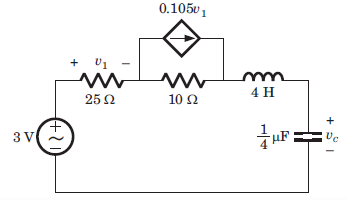Solution: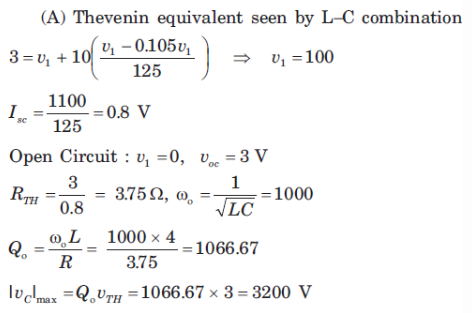QUESTION: 12

Find the resonnant frequency for the circuit.​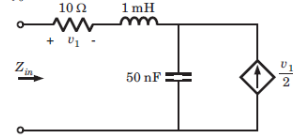Solution: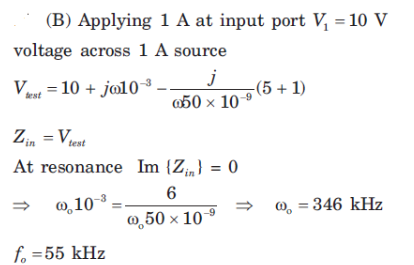QUESTION: 13

Determine the resonant frequency of the circuit.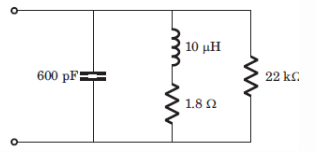Solution: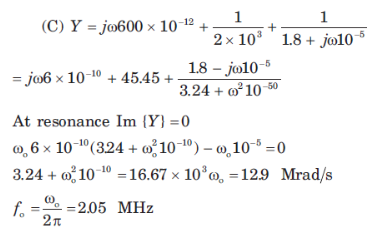QUESTION: 14

The value of C and A for the given network function is​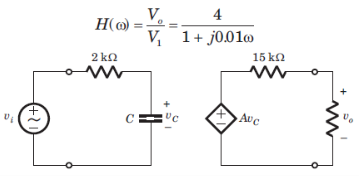Solution: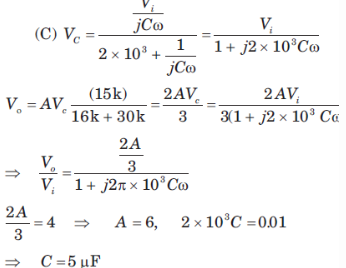QUESTION: 15

H(w) = Vo/Vi = ?​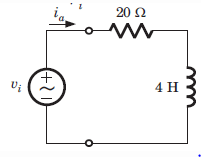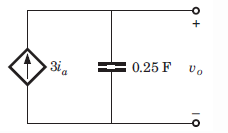Solution: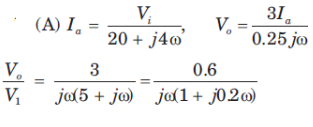QUESTION: 16

H(w) = Vo/Vi = ?​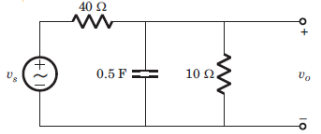Solution: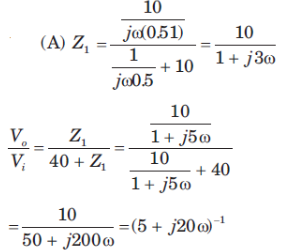QUESTION: 17

The value of input frequency is required to cause a gain equal to 1.5. The value is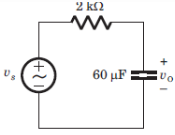Solution: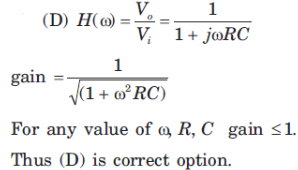QUESTION: 18

In the circuit shown phase shift equal to 45 degrees and is required at frequency w = 20 rad/s. The value of R (in kilo-ohm) is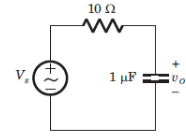Solution: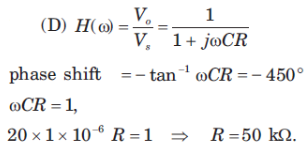QUESTION: 19

For the circuit shown the input frequency is adjusted until the gain is equal to 0.6. The value of the frequency is​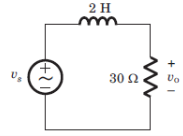Solution: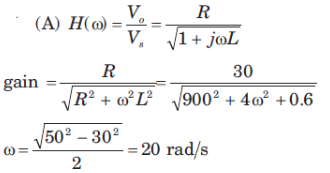QUESTION: 20

For a stable closed loop system, the gain at phase crossover frequency should always be:

Solution:

Phase crossover frequency is the frequency at which the gain of the system must be 1 and for a stable system the gain is decibels must be 0 db.